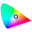#### File Exchange > Graphing >    Chromaticity DiagramAuthor:
OriginLab Technical Support
3/1/2018
Last Update:
1/10/2022
1784
Total Ratings:
35
File Size:
460 KB
Average Rating:File Name:
Chromatici...am.opx
File Version:
1.52
Minimum Versions:
Free
Summary:

Chromaticity diagram template with support for PL data and L*a*b* data.

Description:

PURPOSE
This app can be used to:

1. Open a workbook template with CIE 1931 color space chromaticity diagram and CIE 1976 UCS (uniform chromaticity scale) diagram.
2. Calculate CIE XYZ from photoluminescence spectra and add PL data to the chromaticity diagram.
3. Plot L*a*b* data in 2D, 3D and convert L*a*b* data to CIE 1931 xyY.
4. Convert XYZ to L*a*b*, XYZ to RGB and xyY to XYZ.

INSTALLATION
Download ChromaticityDiagram.opx file, and then drag-and-drop onto the Origin workspace. An icon will appear in the Apps Gallery window.

OPERATION
With a worksheet or a graph active, click the app icon. A toolbar with three buttons will appear.

• Open Chromaticity Diagram Template
Click the first button, and it will open a workbook template. In the third sheet, two graph templates (CIE 1931 and CIE 1976) are inserted. Open the graph window by double clicking on the worksheet's cell. If you want to show color temperature in CIE 1931, click Show/Hide Color Temperature button on the bottom right of the graph. You can also add your own data to the graph template.
• Chromaticity Diagram with PL
1. Click the second button, and it will open the PL2CIE dialog.
2. Choose Phtoluminescence Spectra (PL spectra) from the worksheet or the active graph. You can choose multiple PL spectra, and each PL spectrum corresponds to a point in the chromaticity diagram.
3. In Settings branch, select Spectral Type, two types are available: Radiance and Reflectance or Transmittance (0-1). The latter requires input to be between 0 and 1. For the latter, select Spectral Power Distribution of Illuminant, four options are available, A, D50, D65 and Custom, for the custom type, user should replace PL sheet's column M with his actual SPD data in the app folder's Chromaticity.ogwu file. Select Standard Observer: CIE 1931 2° or CIE 1964 10°, which defines the color matching function.
4. In Graph branch, select CIE type for the diagram. Two options are available: CIE 1931 and CIE 1976. Choose plot type (Scatter or Line+Symbol) and determine whether to show spectrum labels in the graph.
5. Click OK button. A chromaticity diagram with points for PL data will be created. If you want to show color temperature in CIE 1931, click Show/Hide Color Temperature button on the bottom right of the graph. Workbook data for the graph will be hidden. You can activate the workbook in Project Explorer.
• L*a*b* Plot

To see how to create above graphs using the app, right-click the app icon in Apps Gallery window, choose Show Samples Folder from the short-cut menu. Open LABDoc.opju project file.
• 2D Scatter Plot
• 3D Scatter Plot
• 3D Ellipsoid Plot
• Convert CIE 1976 L*a*b* to CIE 1931 xyY
• Converter
Click the fourth Converter button, the converter template will open. It supports to convert XYZ to L*a*b*, XYZ to RGB and xyY to XYZ.Type XYZ values in columns A, B and C, L*a*b* values in columns D, E and F will be calculated automatically. Columns J, K, L and P, Q, R works in the same way.

ALGORITHM

• Chromaticity Diagram with PL
$$X=\int f(\lambda) \bar{x}(\lambda) d\lambda$$, $$Y=\int f(\lambda) \bar{y}(\lambda) d\lambda$$, $$Z=\int f(\lambda) \bar{z}(\lambda) d\lambda$$
where $$f(\lambda)$$ is the spectra, $$\bar{x}(\lambda)$$, $$\bar{y}(\lambda)$$ and $$\bar{z}(\lambda)$$ are CIE's color matching functions.
• Reflectance or Transmittance Spectra
$$X=\frac{1}{N}\int S(\lambda) I(\lambda) \bar{x}(\lambda) d\lambda$$, $$Y=\frac{1}{N}\int S(\lambda) I(\lambda) \bar{y}(\lambda) d\lambda$$, $$Z=\frac{1}{N}\int S(\lambda) I(\lambda) \bar{z}(\lambda) d\lambda$$
where $$S(\lambda)$$ is the spectra, $$I(\lambda)$$ is the spectral power distribution of the illuminant, $$\bar{x}(\lambda)$$, $$\bar{y}(\lambda)$$ and $$\bar{z}(\lambda)$$ are CIE's color matching functions. And $$N=\int I(\lambda) \bar{y}(\lambda) d\lambda$$.

REFERENCE

NOTE

1. PL spectrum's x data represents wavelength, and the units must be nm.
2. In L*a*b* Plot, reference white point is D65, and color space is sRGB.

v1.52 1/7/2022 Replaced background image with image plot of matrix data.
v1.4 12/28/2021 Added a template to convert XYZ to L*a*b*, XYZ to RGB and xyY to XYZ.
v1.3 11/17/2021 Supported reflectance and transmittance spectra.
v1.2 12/18/2020 Supported to plot L*a*b data.
v1.1 1/2/2019 Added a button to show color temperature in CIE 1931 graph template.

06/12/2022郭军Generally, you can scroll the mouse wheel to zoom in/out the whole graph. To scale in/out the graph area, you can set the Rescale from Fxied to Normal in Scale tab of Axis dialog and then use the Scale in/out to zoom the interesting area.
Thanks,
Shirley
OriginLab03/29/2022ddddwangno03/23/2022sreedhar84useful software for graphing01/11/2022william_lin12/22/202178557855to teat

12/17/2021OriginLabHi plexitexi,
Please send us your files(source data file and Origin project file) to let us check the details.
https://www.originlab.com/index.aspx?go=Support/SendFilestoSupport
Thanks,
Shirley
OriginLab

11/30/2021plexitexiThanks for the response and the link to the video. I tried making a file that had my x, y in tab delimited format but it still did not plot the values (it did import them into a book). Any suggestion on what the format of the data.dat file is? thanks so much.

11/30/2021OriginLabHi plexitexi,
You can refer to this video tutorial: https://www.originlab.com/videos/details.aspx?id=223
This App provides chromaticity diagram templates where data can be added to the diagram.

Thanks,
OriginLab

 1 2 3 4